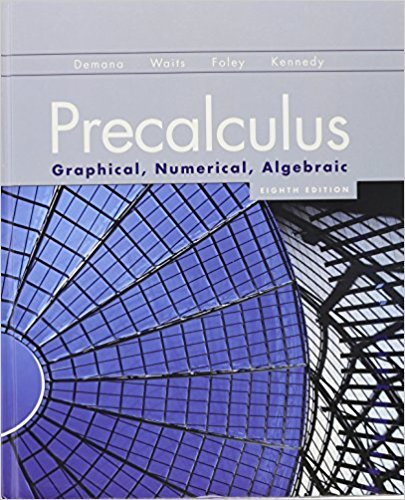×
×

# Solutions for Chapter P.7: Prerequisites## Full solutions for Precalculus: Graphical, Numerical, Algebraic | 8th Edition

ISBN: 9780321656933Solutions for Chapter P.7: Prerequisites

Solutions for Chapter P.7
4 5 0 289 Reviews
26
4
##### ISBN: 9780321656933

Precalculus: Graphical, Numerical, Algebraic was written by and is associated to the ISBN: 9780321656933. This expansive textbook survival guide covers the following chapters and their solutions. Since 58 problems in chapter P.7: Prerequisites have been answered, more than 42138 students have viewed full step-by-step solutions from this chapter. Chapter P.7: Prerequisites includes 58 full step-by-step solutions. This textbook survival guide was created for the textbook: Precalculus: Graphical, Numerical, Algebraic, edition: 8th Edition.

Key Calculus Terms and definitions covered in this textbook
• Arcsine function

See Inverse sine function.

• Binomial probability

In an experiment with two possible outcomes, the probability of one outcome occurring k times in n independent trials is P1E2 = n!k!1n - k2!pk11 - p) n-k where p is the probability of the outcome occurring once

• Conic section (or conic)

A curve obtained by intersecting a double-napped right circular cone with a plane

• Constant term

See Polynomial function

• De Moivre’s theorem

(r(cos ? + i sin ?))n = r n (cos n? + i sin n?)

• Focal length of a parabola

The directed distance from the vertex to the focus.

• Index

• Linear programming problem

A method of solving certain problems involving maximizing or minimizing a function of two variables (called an objective function) subject to restrictions (called constraints)

• Linear regression

A procedure for finding the straight line that is the best fit for the data

• Lower bound for real zeros

A number c is a lower bound for the set of real zeros of ƒ if ƒ(x) Z 0 whenever x < c

• Mode of a data set

The category or number that occurs most frequently in the set.

• Negative association

A relationship between two variables in which higher values of one variable are generally associated with lower values of the other variable.

• Numerical derivative of ƒ at a

NDER f(a) = ƒ1a + 0.0012 - ƒ1a - 0.00120.002

• Outliers

Data items more than 1.5 times the IQR below the first quartile or above the third quartile.

• Quartic function

A degree 4 polynomial function.

• Reflection

Two points that are symmetric with respect to a lineor a point.

• Semimajor axis

The distance from the center to a vertex of an ellipse.

• System

A set of equations or inequalities.

• Variable

A letter that represents an unspecified number.

• Ymin

The y-value of the bottom of the viewing window.

×